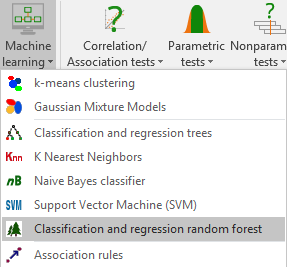# Classification and regression random forests

This powerful machine learning algorithm allows you to make predictions based on multiple decision trees. Set up and train your random forest in Excel with XLSTAT.## What is a Random Forest

Random forests provide predictive models for classification and regression. The method implements binary decision trees, in particular, CART trees proposed by Breiman et al. (1984).

• In classification (qualitative response variable): The model allows predicting the belonging of observations to a class, on the basis of explanatory quantitative and/or qualitative variables.
• In regression (continuous response variable): The model allows to build a predictive model for a quantitative response variable based on explanatory quantitative and / or qualitative variables.

The general principle of the method is to aggregate a collection of predictors (here CART trees) in order to obtain a more efficient final predictor.

## Options for classification and regression random forests in XLSTAT

Two variants are implemented in XLSTAT. Bagging for "Bootstrap aggregating" proposed by Breiman (1996), and Random Input introduced by Breiman in (2001).

Bagging: The idea here is the following: build CART trees from different bootstrap samples, modify the predictions, and so build a varied collection of predictors. The aggregation step allows then to obtain a robust and more efficient predictor.

Random Input Selection: The Random Input variant is an important modification of the bagging. Its objective is to increase the independence between the models (trees), in order to obtain a final model with better performance.

The following options are proposed to configure the set-up of a random forest within XLSTAT:

Sampling method: Observations are chosen randomly and may occur only once or several times in the sample.

Sample size: Enter the size k of the sample to generate for the tree's construction.

Number of trees: Enter the desired number of trees q in the forest.

Tree parameters:

• Minimum parent size: Enter the minimum number of observations that a node must contain to be split.
• Minimum child size: Enter the minimum number of observations that every newly created node must contain after a possible split in order to allow the splitting.
• Maximum depth: Enter the maximum tree depth.

Stop conditions:

• Complexity parameter (classification only): Enter the value of the complexity parameter (CP). The construction of a tree does not continue unless the overall impurity is reduced by at least a factor CP. That value must be less than 1.
• Construction time (in seconds): Enter the maximum time allowed for the construction of all trees in the forest. Past that time, if the desired number of trees in the forest could not be built, the algorithm stops and returns the results obtained using the trees built until then.

## Results for classification and regression random forests in XLSTAT

OOB error: Activate this option to display the Out-Of-Bag error of the forest.

OOB predictions: Activate this option to display the vector of Out-Of-Bag predictions.

OOB predictions details: Activate this option to display OOB predictions details

OOB times: Activate this option to display for each observation of the learning sample the number of times it was OOB.

Confusionmatrix (classification only): Activate this option to display the table showing the numbers of well- and badly-classified observations for each of the categories.

OOB error evolution: Activate this option to display the table showing the evolution of the OOB error according to the number of trees.

Variable importance: The importance measure for a given variable is the mean error increase of a tree when the observed values of this variable are randomly exchanged in the OOB samples.### analyze your data with xlstat

14-day free trial

Included in